Home Standards English Math Links Games
MATH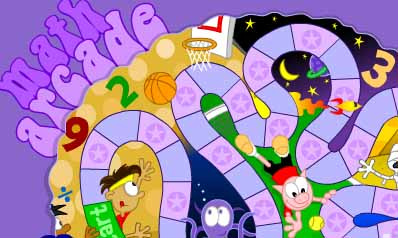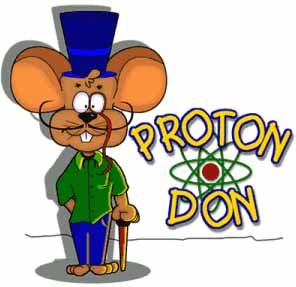øAwesome Facts PowerpointsMath Soccer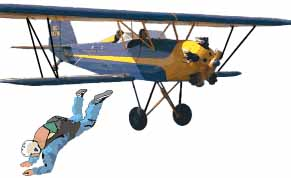Line Jumper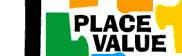Place Value Puzzler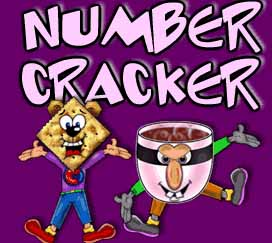Number CrackerSoccer ShootoutShape Surveyor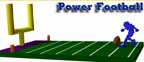PowerFootball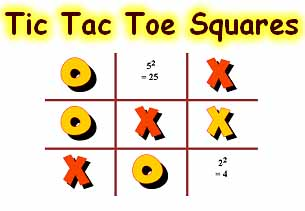Tic Tac Toe Squares Space Fractions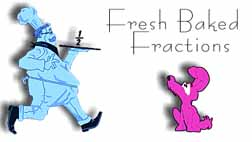Fresh Baked Fractions

 Easy Games Starmatica Probability BLACK LINE MASTERS Rounding Video Operation Order Solving Equations Video Word Problems by grade Multiplication practice Word Problems Test On line Word Problems Interactive On line Word Problems all levels Probability Spinners MATH GRAPHS Probability Number Spinner

 Words for Addition Words for Subtraction Words for Multiplication Words for Division total sum add up to in all together both increased by all together in all difference less than decreased by left over remain take away have left fewer than how much more how many more how much less how many less change (in money problems) times how many times a product of double triple groups (boxes, dozens, packets) three times as many three times as much twice as many twice as much as divided by average half as much any fraction parts equal parts unit cost sharing split up or cut up equally distributed how many... on each

Test addtion, subtraction & division 1 minute eachAddition WorksheetsSubtraction Worksheets Test Add-subtr-divide1Multiplication Worksheets Test all 1 minute per lineDivision Worksheets Skip countCreate Multipications WorkshetsMultiplication InteractiveSuper teacher Multiplication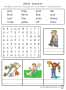Multiplication Printables Create your own Page Math is Fun Interactive times table 3rd grade worksheetsMATH HELPMATH THIS WEEKFACTIONS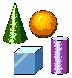GEOMETRY MATH FACTS FRACTION SHEETS GRAPHS FREE TESTS

MATH GAMESMultiplication GameAddition GameHidden Picture SubtractionDivision GameHidden Picture AdditionHidden picture DivisionPlanet Blaster Add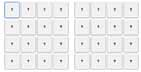Concentration AdditionConcentration Multiplication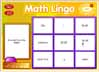Math Lingo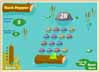Rock Hopper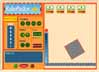Robo PackerInternet Algebra Math Flashcards Homework Help Woksheets Math Games Math Graphs Sites DIVISION DECIMALS FRACTIONS

 Rounding Cadence (T) Find the place you�re rounding to  (Kids repeat) (T) Look next door check what to do (Kids repeat) (T) For the numbers 5 through 9 (Kids repeat) (T) Round that number up the line (Kids repeat) (T) For the numbers 0 through 4 (Kids repeat) (T) Round it down, do nothing more (Kids repeat) (T) Round up (Kids) 5 through 9 (T) Round down (Kids) 0 through 4 (Together) Rounding numbers it�s so ... easy

MATH SITES

 1st Grade Math 2nd Grade Math 3rd Grade Math 4th Grade Math 5th Grade Math Math Exchange Algebra Worksheets Math Help Ask Dr. Math Today's Challenge Make Math Pages Fractins & Percent Fraction Worksheets Fractions & Decimals Fractions & Percent Quiz Fractions & Percent Worksheet Elementary Math Create a Graph Divisibility Rules On line Math Test Math Lessons Geobard Chalenginh Problems On line practice Word Problems Math Activities Math Strategies Math Resources Mathematics Skills 1 Mathematics Skills 2 Mathematics Skills 3 Mathematics Skills 4 TOTAL MATH ALGEBRA DR. MATH TODAY'S CHALLENGE BASE 10 BLOCK Divisibility rules Time, Money, Measurement MATH ALL CLOCK WORD PROBLEMS Clock & time games Calculate Time Teaching time Math Tests Math Lessons HM Math Inverse Operations Word problems Math On Line Math Activities Printables Homework Help Teaching Help Math Gossary Sites Collection Lesson Ideas Centers Can Teach Problem Solving Animated Dictionary More Activities Math Solutions MathSteps 3 Math Stories Worksheets Generator Math Worksheets Mental Math Money Practice Money Instructor Money Problems Fun Fractions Math Topics Multiplication Flash Cards Owesome On line Math Games Saxson on line Coordenates Arihtmetic Problem Solving Translate a problem Bananas problem Legs problem Tangrams Time worksheet creator WWWMath WebMath Time, Money, Measurement Visual Fractions Worksheets 1 Worksheets 2 Worksheets 3 Worksheets 4

MATH ENRICHMENT
MATH IDEAS

MATH STANDARDS TESTING PER GRADE LEVEL